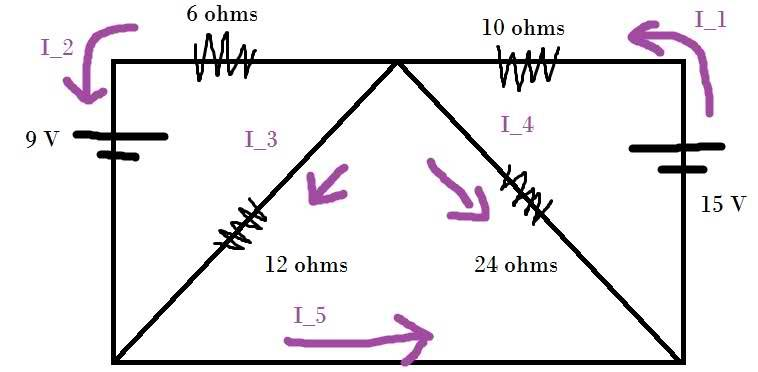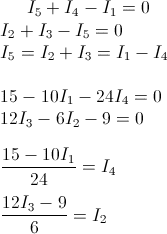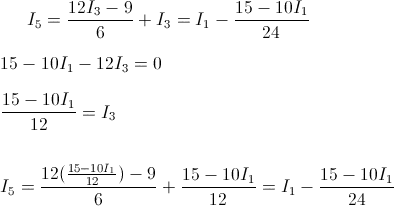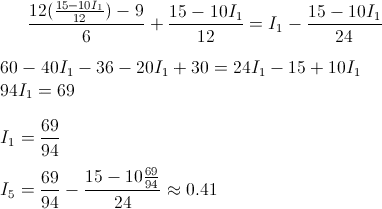# Calculating current in a wire

## Homework Statement

Find the current in the bottom-most wire (the I5 current).## Homework Equations

$$I=\frac{\Delta V}{R}$$
Kirchhoff's current and loop rules.

## The Attempt at a Solution

Using Kirchoff's current and loop rules, I obtained:I then substitued my results into my equation for I5 and used Kichoff's rules again to put I5 in terms of I1Then I solved for I1 and used that to solve for I5.Unfortunately, the answer in my book is 2.1 amps. I've worked this problem multiple times, and the only thing worse than doing all that algebra over and over again is getting a wrong answer each time. Can someone help me out here?

Also, I didn't see an intro section, so I guess I'll take care of that here. My handle is BadAnima, and I'm currently a freshman in university. My major is chemical engineering, but I'm not sure if I want to stay on this track or go into physics.

Redbelly98
Staff Emeritus
Homework Helper
Welcome to Physics Forums.

I would go ahead and find all 5 currents. Then check for consistency in the original two KCL equations and 3 KVL loops equations. (i.e., do your values satisfy I5+I4-I1=0, etc.)

That way you can convince yourself whether the book or your answer is wrong, and go from there. Post back with what you find, I'll be online from time to time throughout today (Eastern USA time) if you need more help.

p.s. I did not find any algebra mistake, but then again there is a lot of algebra so I could be missing the same thing you are. Or the book could be wrong.

gneill
Mentor
I then substitued my results into my equation for I5 and used Kichoff's rules again to put I5 in terms of I1The simplification for I3 looks a bit dodgy. Better check it.

p.s. I did not find any algebra mistake, but then again there is a lot of algebra so I could be missing the same thing you are. Or the book could be wrong.

Before I rework the problem again, I have the solution manual entry for this problem. Would it help to post that instead?
The simplification for I3 looks a bit dodgy. Better check it.

I don't see where I went wrong with it. I just traveled around another loop in the circuit.

gneill
Mentor
I don't see where I went wrong with it. I just traveled around another loop in the circuit.

Ah. My bad. I thought you'd solved the equation above it for I3. I guess I expected all the loop equations to be written together, not have another pop up partway through.

If it's any consolation, I solved for I5 using mesh equations and arrived at a result that matches yours, namely 39/94 ≈ 0.415 amps.

Redbelly98
Staff Emeritus
Homework Helper
Before I rework the problem again, I have the solution manual entry for this problem. Would it help to post that instead?
Not yet, at this point. I think it would be more useful to learn how to verify (and/or debug) your algebra. So: solve for all unknown quantities, then verify whether their values satisfy the original equations you wrote to begin solving the problem.

Not yet, at this point. I think it would be more useful to learn how to verify (and/or debug) your algebra. So: solve for all unknown quantities, then verify whether their values satisfy the original equations you wrote to begin solving the problem.

Okay, I found all of the currents individually and checked my equations. They all sum to zero as they should. I'm starting to think that the book is wrong. It wouldn't be the first time.

Redbelly98
Staff Emeritus